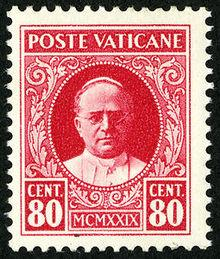# Three-euro 6035

The boy bought stamps worth € 2 and € 3 worth, a total of € 65. Two euros was five times more than three-euro. How many marks were there?

a =  25
b =  5

### Step-by-step explanation:

2a+3b = 65
a = 5b

2·a+3·b = 65
a = 5·b

2a+3b = 65
a-5b = 0

Row 2 - 1/2 · Row 1 → Row 2
2a+3b = 65
-6.5b = -32.5

b = -32.5/-6.5 = 5
a = 65-3b/2 = 65-3 · 5/2 = 25

a = 25
b = 5

Our linear equations calculator calculates it.Did you find an error or inaccuracy? Feel free to write us. Thank you!

Tips for related online calculators
Do you have a system of equations and looking for calculator system of linear equations?